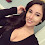## Thursday 17 October 2013

### Paired Student's t test Calculator

The Paired Student's t-test is a parametric test that determines whether two groups of matched (i.e. dependent) samples are the same. It thus requires that the two groups have the same number of samples. For example, we could have the blood pressure readings for a group of patients before a certain medication is applied, and then the corresponding blood pressure readings for the same group of patients a few months after treatment. If there are $N$ readings, samples 1 of the two datasets will be the reading from Patient 1 before and after treatment, samples 2 will be the readings from Patient 2 before and after treatment, and so on. A worked example of carrying out the Paired Student's t-test can be found here

Below is an online calculator that implements the Paired Student's t-test. Please enter Group 1 and Group 2 values as comma separated numbers in the fields below. Group 1 and Group 2 must have the same number of samples. Alternatively, you can choose a two-column CSV file to load - simply press on the choose file button below the clear Group 1 and Group 2 buttons. To reload the same file after clearing the text areas, you would need to reload this webpage.

At the bottom there is a graph that will display the histograms of both the groups and their difference. This conveys a lot of useful information visually. As we assume that all the data are distributed Normally, the spread of the histograms will be proportional to the variance of the samples within the groups, and the centre of the histograms will coincide with the means of the samples in the groups. The greater the mean of the group differences, and the lower the variance of the differences, the higher the calculated t value will be, leading to a lower p-value, possibly yielding a significant result. The histogram of the differences is key to seeing this - for a low p-value, it will be very narrow, and will be centred on a noticeably large value of the mean. As for the two groups' histograms, they will be widely spaced apart, with very similar spreads, indicating a systematic difference.

 Group 1 values: Group 2 values:

Results pending...

 Histograms of the two groups Frequency Values
 Histogram of differences Frequency Values

1.2.